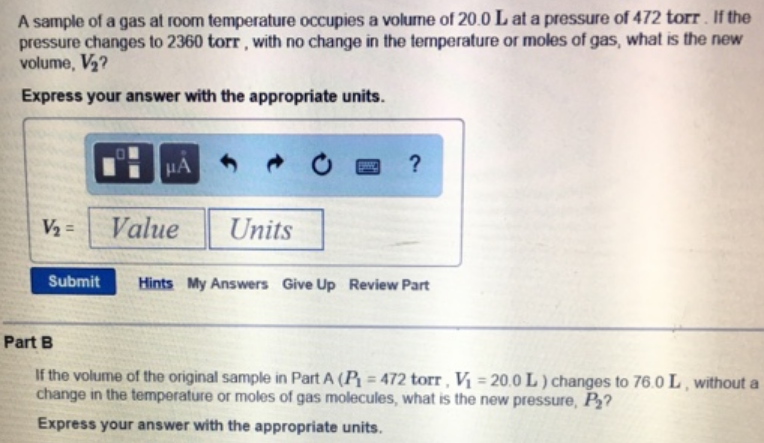# Problem: A sample of a gas at room temperature occupies a volume of 20.0 L at a pressure of 472 torr. If the pressure changes to 2360 torr, with no change in the temperature or moles of gas, what is the new V2? Express your answer with the appropriate units. If the volume of the original sample in Part A (P1 = 472 torr, V1 = 20.0 L) changes to 76.0 L, without a change in the temperature or moles of gas molecules, what is the new pressure, P2? Express your answer with the appropriate units.

###### FREE Expert Solution
83% (87 ratings)###### Problem Details

A sample of a gas at room temperature occupies a volume of 20.0 L at a pressure of 472 torr. If the pressure changes to 2360 torr, with no change in the temperature or moles of gas, what is the new V2? Express your answer with the appropriate units. If the volume of the original sample in Part A (P1 = 472 torr, V1 = 20.0 L) changes to 76.0 L, without a change in the temperature or moles of gas molecules, what is the new pressure, P2? Express your answer with the appropriate units.